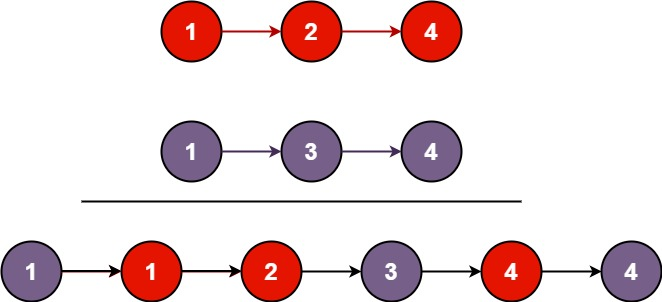| English | 简体中文 |

# 21. Merge Two Sorted Lists

## Description

You are given the heads of two sorted linked lists list1 and list2.

Merge the two lists in a one sorted list. The list should be made by splicing together the nodes of the first two lists.

Example 1:Input: list1 = [1,2,4], list2 = [1,3,4]
Output: [1,1,2,3,4,4]


Example 2:

Input: list1 = [], list2 = []
Output: []


Example 3:

Input: list1 = [], list2 = 
Output: 


Constraints:

• The number of nodes in both lists is in the range [0, 50].
• -100 <= Node.val <= 100
• Both list1 and list2 are sorted in non-decreasing order.